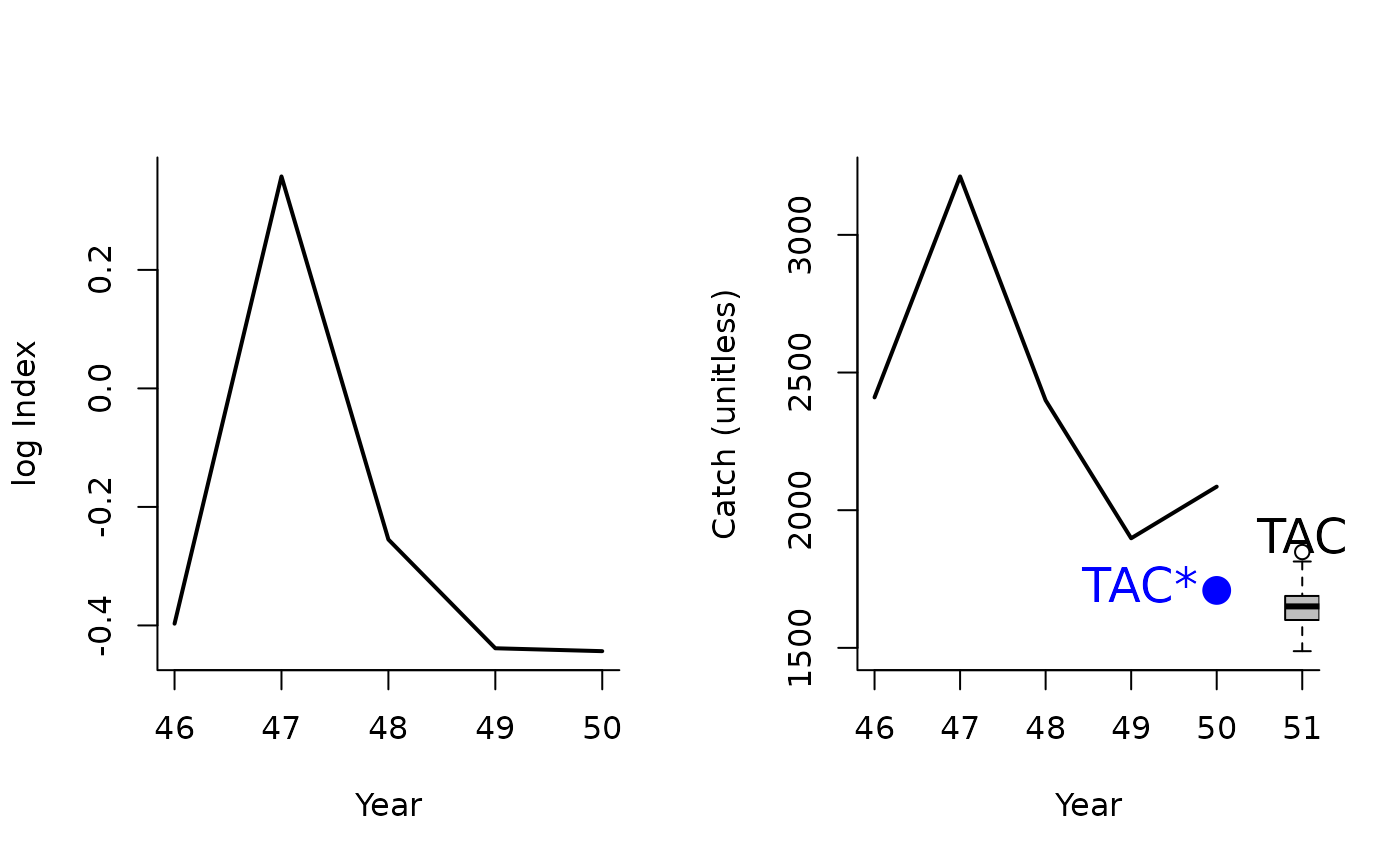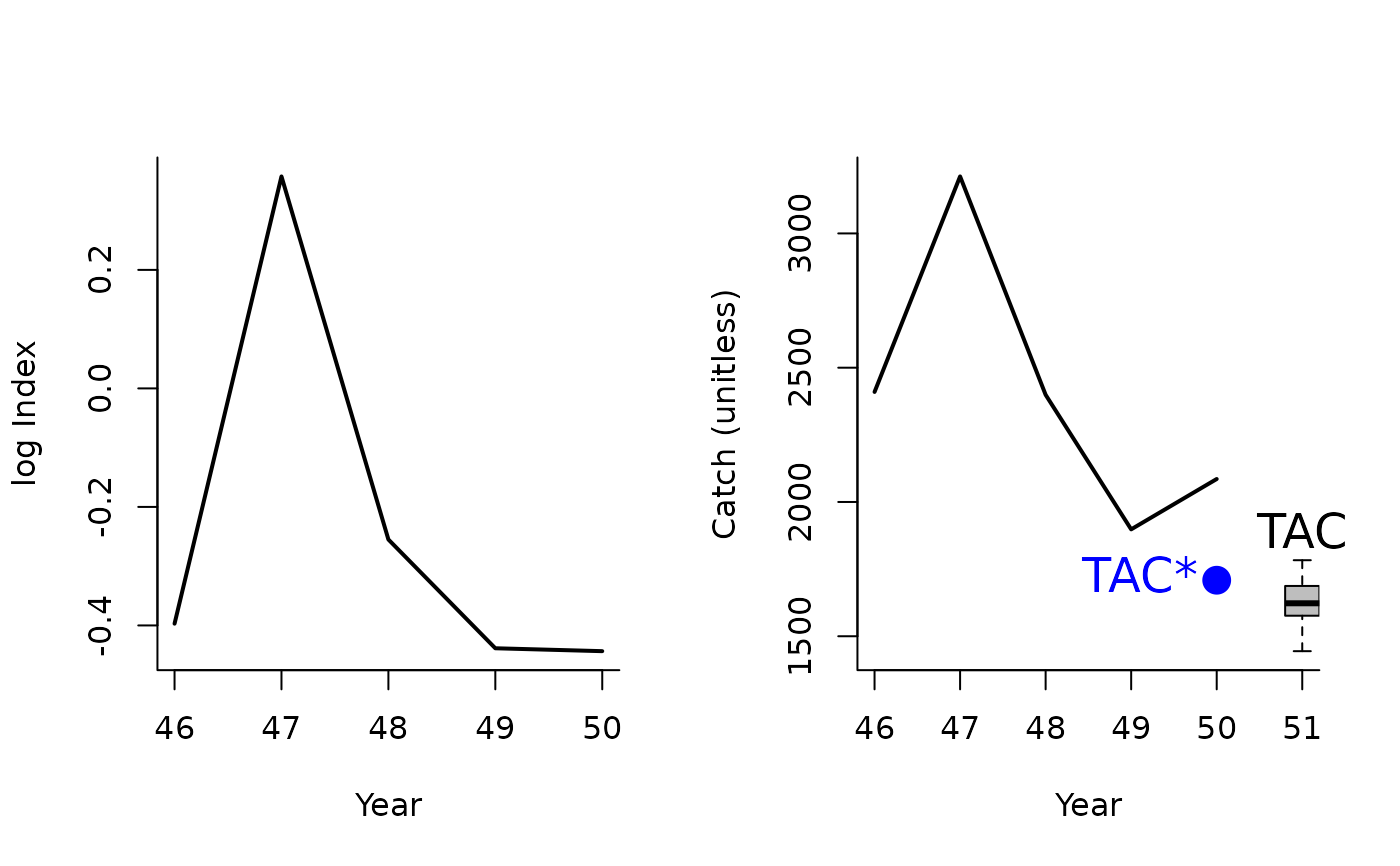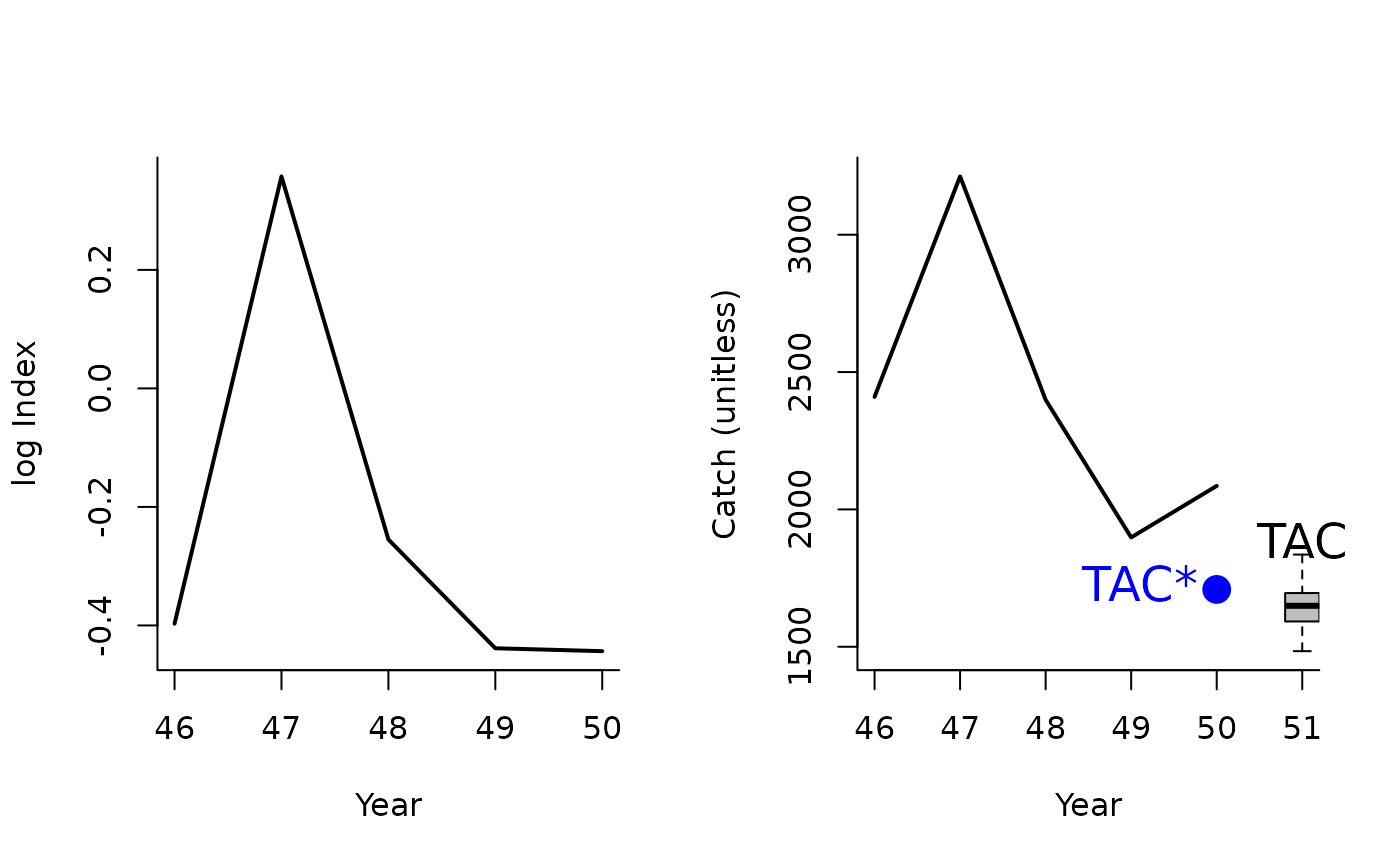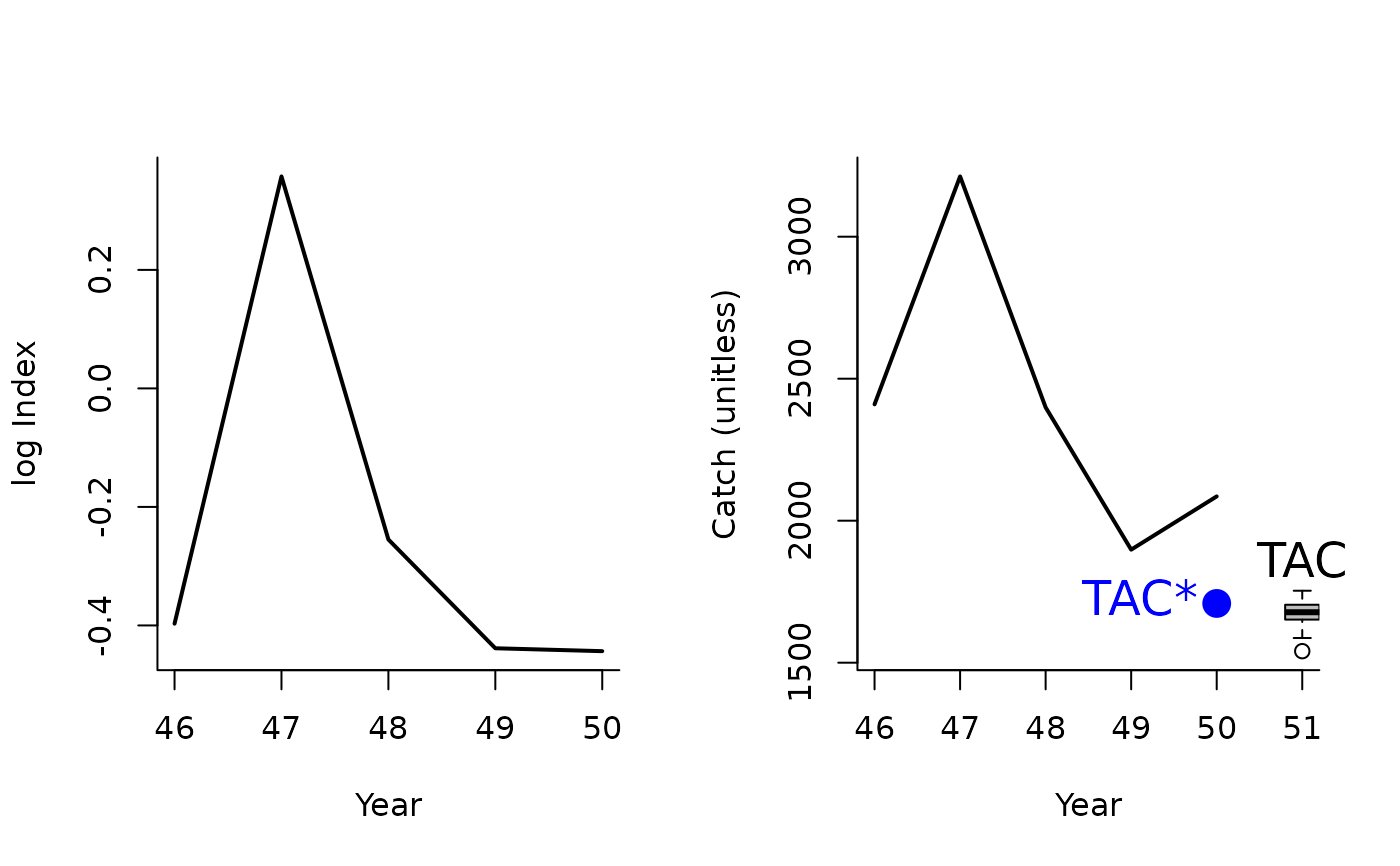A management procedure that incrementally adjusts the TAC to maintain a constant CPUE or relative abundance index.

Islope1(x, Data, reps = 100, plot = FALSE, yrsmth = 5, lambda = 0.4, xx = 0.2)

Islope2(x, Data, reps = 100, plot = FALSE, yrsmth = 5, lambda = 0.4, xx = 0.3)

Islope3(x, Data, reps = 100, plot = FALSE, yrsmth = 5, lambda = 0.4, xx = 0.4)

Islope4(x, Data, reps = 100, plot = FALSE, yrsmth = 5, lambda = 0.2, xx = 0.4)

## Arguments

x

A position in the data object

Data

A data object

reps

The number of stochastic samples of the MP recommendation(s)

plot

Logical. Show the plot?

yrsmth

Years over which to calculate index

lambda

A gain parameter controlling the speed in update in TAC.

xx

Parameter controlling the fraction of mean catch to start using in first year

## Value

An object of class Rec-class with the TAC slot populated with a numeric vector of length reps

## Details

The TAC is calculated as: $$\textrm{TAC} = \textrm{TAC}^* \left(1+\lambda I \right)$$ where $$\textrm{TAC}^*$$ is $$1-xx$$ multiplied by the mean catch from the past yrsmth years for the first year and catch from the previous year in projection years, $$\lambda$$ is a gain parameter, and $$I$$ is the slope of log index over the past yrsmth years.

## Functions

• Islope1: The least biologically precautionary of the Islope methods

• Islope2: More biologically precautionary. Reference TAC is 0.7 average catch

• Islope3: More biologically precautionary. Reference TAC is 0.6 average catch

• Islope4: The most biologically precautionary of the Islope methods. Reference TAC is 0.6 average catch and gain parameter is 0.2

## Required Data

See Data-class for information on the Data object

Islope1: Cat, Ind, LHYear, Year

## Rendered Equations

See Online Documentation for correctly rendered equations

Other Index methods: GB_slope(), GB_target(), Gcontrol(), ICI(), Iratio(), Itarget1_MPA(), Itarget1(), ItargetE1()

T. Carruthers

## Examples

Islope1(1, MSEtool::SimulatedData, plot=TRUE)#> TAC (median)
#>     1639.435
Islope2(1, MSEtool::SimulatedData, plot=TRUE)#> TAC (median)
#>     1651.995
Islope3(1, MSEtool::SimulatedData, plot=TRUE)#> TAC (median)
#>     1656.359
Islope4(1, MSEtool::SimulatedData, plot=TRUE)#> TAC (median)
#>     1668.957